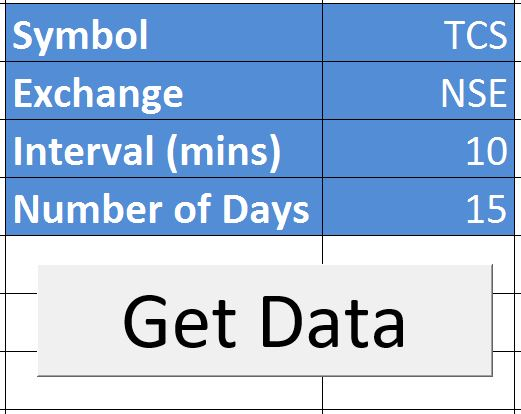### On Balance Volume (OBV) Trading Strategy

Discover a potent momentum indicator – the On Balance Volume (OBV) Trading Strategy. Learn how to use it for predicting price movements. Dive into the world of OBV with insights on calculating and interpreting it. Plus, access a real-time Excel sheet for comprehensive stock analysis.

Before we delve into the OBV Trading Strategy, check out our selection of highly effective Excel-based trading systems:

### Calculating the OBV Trading Strategy

Unlock the power of the OBV Trading Strategy by understanding its calculations:

• The initial OBV in the price series equals the absolute volume.
• If the current price surpasses the previous price, calculate the current OBV by adding the current volume to the previous OBV.
• If the current price is lower than the previous price, calculate the current OBV by subtracting the current volume from the previous OBV.
• If the current price matches the previous price, the current OBV remains unchanged.

### The Theory Behind the OBV Trading Strategy

Delve into the underlying theory of the OBV Trading Strategy and understand how it tracks the smart money flow in the market. Discover why significant volume changes without substantial price shifts signal future price movements. Gain insights into how institutional investors’ actions influence this indicator.

### Interpreting the OBV Trading Strategy

Interpret the OBV Trading Strategy effectively. Learn to identify bullish and bearish divergences. Understand how to use OBV in conjunction with other price action patterns for well-informed trading decisions.

### On Balance Volume Trading Strategy Excel Sheet Overview

Discover a user-friendly Excel sheet that simplifies the process of calculating OBV, change in OBV (%), and change in price (%). Just enter the stock name, exchange, and timeframe, and let the Excel sheet handle the rest.

Take a look at the screenshots below:### How to Use the On Balance Volume Trading Strategy Excel Sheet

Follow these simple steps to effectively use the Excel sheet:

• Step 1: Download the Excel file from the end of this post.
• Step 2: Open the Excel file while connected to the internet. Accept any prompts to enable Macros and Data connections if they appear.
• Step 3: Input the Symbol Name, Exchange Name, Interval, and Number of Days.
• Step 4: Click the “Get Data” button. The data will be automatically downloaded, and OBV values will be calculated. This data can be obtained for a maximum of 15 days, with a minimum interval of 1 minute.dexterDecember 5, 2023dexterSeptember 20, 2023dexterOctober 13, 2023